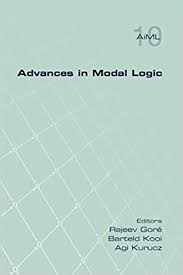Haoyu Wang, Yanjing Wang, Yunsong Wang
Publication year: 2022

In this paper, we present an epistemic interpretation of the tensor disjunction in dependence logic, inspired by the weak disjunction studied by Medvedev under a formalized BHK-like semantics. To put the tensor disjunction in the same picture with intuitionistic and classical disjunctions, we give an alternative epistemic semantics to inquisitive logic with tensor, studied by Ciardelli and Barbero (2019). We then use a much more powerful epistemic language with propositional quantifiers to give a complete axiomatization where the non-classical formulas are reduced in the system to classical but epistemic ones. It also turns out that the idea of the tensor disjunction can be generalized greatly without adding expressive power, resulting in a (k, n)-parametrized general tensor that captures the epistemic state that knowing k out of n given answers to the corresponding n questions are correct. The standard tensor is simply a special case where k=1 and n=2.